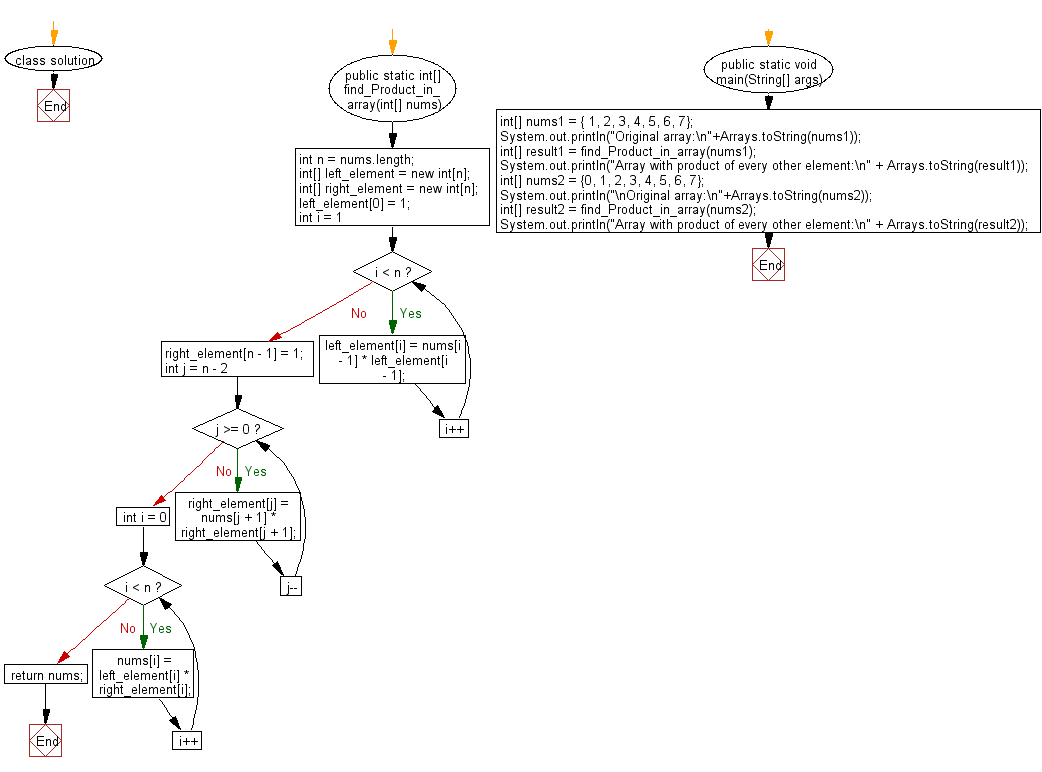﻿ Java: Replace each element of the array with its product# Java Array Exercises: Replace each element of the array with product of every other element in a given array of integers

## Java Array: Exercise-63 with Solution

Write a Java program to replace each element of the array with product of every other element in a given array of integers.

Example:
Input :
nums1 = { 1, 2, 3, 4, 5, 6, 7}
nums2 = {0, 1, 2, 3, 4, 5, 6, 7}
Output:
Array with product of every other element:
[5040, 2520, 1680, 1260, 1008, 840, 720]
Array with product of every other element:
[5040, 0, 0, 0, 0, 0, 0, 0]

Sample Solution:

Java Code:

``````import java.util.Arrays;

class solution
{
public static int[] find_Product_in_array(int[] nums)
{
int n = nums.length;

int[] left_element = new int[n];
int[] right_element = new int[n];

left_element = 1;
for (int i = 1; i < n; i++) {
left_element[i] = nums[i - 1] * left_element[i - 1];
}

right_element[n - 1] = 1;
for (int j = n - 2; j >= 0; j--) {
right_element[j] = nums[j + 1] * right_element[j + 1];
}

for (int i = 0; i < n; i++) {
nums[i] = left_element[i] * right_element[i];
}
return nums;
}

public static void main(String[] args)
{
int[] nums1 = { 1, 2, 3, 4, 5, 6, 7};
System.out.println("Original array:\n"+Arrays.toString(nums1));
int[] result1 = find_Product_in_array(nums1);
System.out.println("Array with product of every other element:\n" + Arrays.toString(result1));

int[] nums2 = {0, 1, 2, 3, 4, 5, 6, 7};
System.out.println("\nOriginal array:\n"+Arrays.toString(nums2));
int[] result2 = find_Product_in_array(nums2);
System.out.println("Array with product of every other element:\n" + Arrays.toString(result2));

}
}
```
```

Sample Output:

```Original array:
[1, 2, 3, 4, 5, 6, 7]
Array with product of every other element:
[5040, 2520, 1680, 1260, 1008, 840, 720]

Original array:
[0, 1, 2, 3, 4, 5, 6, 7]
Array with product of every other element:
[5040, 0, 0, 0, 0, 0, 0, 0]
```

Flowchart:Java Code Editor:

Improve this sample solution and post your code through Disqus

What is the difficulty level of this exercise?

Test your Programming skills with w3resource's quiz.

﻿

## Java: Tips of the Day

countOccurrences

Counts the occurrences of a value in an array.

Use Arrays.stream().filter().count() to count total number of values that equals the specified value.

```public static long countOccurrences(int[] numbers, int value) {
return Arrays.stream(numbers)
.filter(number -> number == value)
.count();
}
```

Ref: https://bit.ly/3kCAgLb